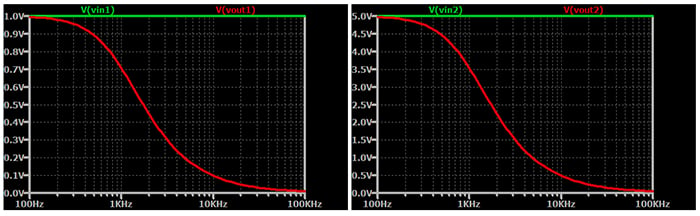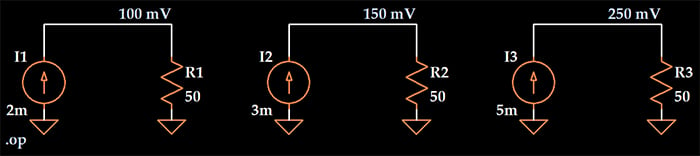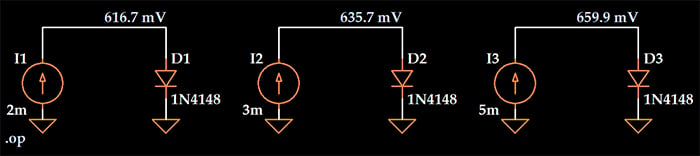Technical Article

# What Is a Linear System?

June 17, 2020 by Robert Keim

## This article explains the essential aspects of linear systems, which play a fundamental role in electrical engineering and signal processing.

### What Is a System?

First things first. For our purposes, a system is a component or collection of components that accepts an input signal and produces an output signal. These components can be physical devices, such as resistors and transistors, or computational processes, such as addition and multiplication. A system can be something as simple as an RC low-pass filter or as complex as a microprocessor.

### The Importance of Linear Systems

Systems that fall into the “linear” category are of special interest for engineers. We have powerful mathematical tools that help us to analyze systems that are both linear and time-invariant, and many physical phenomena can be accurately modeled as linear systems—even though these phenomena are usually not perfectly linear when we account for all the details.

In circuit design, we often strive for linearity because it leads to desirable output characteristics. For example, linear audio amplifiers produce sound with less distortion, and linear operation in an RF signal chain helps a receiver to correctly decode quadrature-amplitude-modulated (QAM) waveforms.

Linearity is the key to mathematical analysis and manipulation in signal-processing applications: a concept known as superposition is the foundation of digital signal processing, and superposition is applicable only when we’re working with linear systems.

### Requirements for Linear Systems

To determine if a system is linear, we need to answer the following question: When an input signal is applied to the system, does the output response exhibit homogeneity and additivity? If a system is both homogeneous and additive, it is a linear system.

#### Homogeneity

Let’s say we apply an input signal x(t) to a system, and it produces an output signal y(t). Then, we apply a signal kx(t), where k is a constant. If the system is linear, the new output signal will be ky(t). If this same thing happens for any input signal and any constant, the system exhibits homogeneity.

The principle of homogeneity is also called the scalar rule or the scaling property. The fundamental idea is that scaling the input signal (i.e., multiplying it by a constant) will scale the output in the same way.

Let’s consider an RC low-pass filter. The amplitude of the output signal depends on the frequency of the input signal, but scaling the input signal will always result in equal scaling of the output signal, regardless of frequency. You can multiply the input signal by any factor and the output signal will be multiplied by the same factor. Thus, the system is homogeneous.##### The output response of a low-pass filter, which is a linear system, scales with the input signal.

Now let’s imagine that our system is a resistive heater. The input signal, x(t), is a current produced by a current source, and the output signal, y(t), is the heat generated by the resistive element. The power dissipated as heat is proportional to the square of the amount of current flowing through the resistor. Thus, if we increase the current by a factor of ten (i.e., k = 10), the output increases by a factor of one hundred. In this case, kx(t) ≠ ky(t), and the system is not homogeneous.

The example of the resistive heater is interesting because most of us, I think, would naturally identify a resistor as a linear component. But it’s not really the component itself that is linear—rather, the relationship between voltage and current, which we call Ohm’s law, is linear. When we’re interested in the relationship between current and power (or voltage and power), the resistor is a nonlinear system.

If the input delivered to an additive system is a composite signal created by adding separate signals, the output will also be a composite signal containing modified versions of these added signals. Another way to think about this is that the system treats the signals that preceded the summation as though they are separate, despite the fact that they are delivered and processed as one signal.

Additivity might be more easily explained via math. Let’s say that the input signals x1(t) and x2(t) produce output signals y1(t) and y2(t). Now, we create a composite signal xC(t) by adding the original two input signals: xC(t) = x1(t) + x2(t). In an additive system, if the input is xC(t), the output yC(t) is the addition of the two original output signals: yC(t) = y1(t) + y2(t).

Let’s say that our system is a 50 Ω resistor; the input signal is a current and the output signal is a voltage. If the input is 2 mA, the output is 100 mV. If the input is 3 mA, the output is 150 mV. If the input is 5 mA (= 2 mA + 3 mA), the output is 250 mV (= 100 mV + 150 mV). Thus, the system is additive.Now let’s drive our input signal through a diode instead of a resistor. The LTspice results shown below demonstrate that when the input signal is a current and the output signal is a voltage, a diode is not an additive system.### Conclusion

We’ve seen that linearity is an important characteristic of engineered systems, and we’ve examined the two properties that separate linear systems from nonlinear systems. However, we need to cover a few more concepts in order to explore this topic more thoroughly; to that end, we’ll discuss time invariance (also called shift invariance), impulse response, static linearity, and sinusoidal fidelity in a future article.